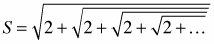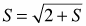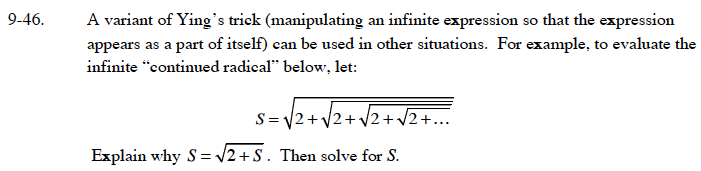### Home > CALC > Chapter 9 > Lesson 9.1.3 > Problem9-46

9-46.

A variant of Ying's trick (manipulating an infinite expression so that the expression appears as a part of itself) can be used in other situations. For example, to evaluate the infinite "continued radical" below, let: Homework Help ✎Explain why. Then solve for S.$S-2=0\text{ or }S+1=0$

Which sum makes sense?